• 绘制阶跃响应曲线的matlab程序，在自控、信号处理等课程中往往可能会用到，比较通俗易懂，方便学习~重新更新了一下资源，可以直接运行，这次注释得很详细！之前下载的同学请重新下载，谢谢！
• 利用MATLAB绘制二阶控制系统的单位阶跃响应曲线 作者张宇涛 张怀超 陈佳伟 一课设目的和意义 学习控制系统的单位阶跃响应 记录单位阶跃响应曲线 比较阻尼比zeta为不同值时曲线的变化趋势 掌握二阶系统时间响应分析的...
• matlab阶跃响应曲线代码自主控制系统 ACS的Matlab代码 文件名 备忘录 页 拉普拉斯变换系数 22 partfrac() 23 拉普拉斯变换laplace() ilaplace() 27-30 ilaplace() ， pretty() ， limit() 31 传递函数tf() ，线性...
• 利用MATLAB绘制二阶控制系统的单位阶跃响应曲线 作者张宇涛 张怀超 陈佳伟 一课设目的和意义 学习控制系统的单位阶跃响应 记录单位阶跃响应曲线 比较阻尼比zeta为不同值时曲线的变化趋势 掌握二阶系统时间响应分析的...
• MATLAB中对象阶跃响应曲线的实现方法：包括各种纯滞后环节的阶跃响应曲线
• matlab阶跃响应曲线代码神经同步，以使用MiRo检测和分类触觉行为 MiRo是一种小型宠物式机器人，旨在作为伴侣。 在其头部和背部设有触觉传感器矩阵。 这些触觉传感器的值是通过基于ROS的用户通信通道获取的。 该任务...
• 将二阶系统的单位阶跃响应 输入后显示 错误: 表达式无效。调用函数或对变量进行索引时，请使用圆括号。否则，请 检查不匹配的分隔符。 以及后面的各种下标，演示应该怎么打？ 刚学matlab，求解答matlab
• matlab阶跃响应曲线代码信号完整性 ＃这是学习和交换信号完整性知识的网站。 适用于信号完整性的Python / Matlab编码涉及30多个项目。 包括： Tx-FIR抽头优化（ZF，MMSE）。 正/负阶跃/脉冲响应分析tran＆statistic...
• 阶跃响应曲线辨识传递函数的图解方法 由阶跃响应曲线辨识传递函数的图解方法
• 2 2 利用 MATLAB 绘制二阶控制系统的单位阶跃响应曲线 作者张宇涛 张怀超 陈佳伟 一课设目的和意义 学习控制系统的单位阶跃响应 记录单位阶跃响应曲线 比较阻尼比 zeta 为不同值时曲线的变化趋势 掌握二阶系统时间...
• 最小相位环节有下列七种： 拉氏变换原理讲解参考：【控制】拉普拉斯拉氏变换原理分解理解 Num Name F(s) f(t) 1 比例环节 K (K>...1T∗e−tT\frac{1}{T}*e^{-\frac{t}{T}}T1​∗e−Tt​
最小相位环节有下列七种： 拉氏变换原理讲解参考：【控制】拉普拉斯拉氏变换原理分解理解
NumNameF(s)f(t)1比例环节

K

(

K

>

0

)

K\ (K>0)

δ

(

t

)

\delta(t)

2惯性环节

1

T

s

+

1

(

T

>

0

)

\frac{1}{Ts+1}\ (T>0)

1

T

∗

e

−

t

T

\frac{1}{T}*e^{-\frac{t}{T}}

3一阶微分环节

T

s

+

1

Ts+1

=

=

4振荡环节

1

s

2

ω

n

2

\frac{1}{\frac{s^2}{\omega_n^2}}

=

=

5二阶微分环节6积分环节

1

s

\frac{1}{s}

1

(

t

)

1(t)

7微分环节

s

s

d

i

r

a

c

(

1

,

t

)

dirac(1, t)

非最小相位环节共有五种：
From: 自动控制原理第6版-P184
展开全文算法
• “使用K-Mean均值算法进行聚类只是一种判断干扰因素的手段，不是最终目的”近期有几个特别热衷于调参的小伙伴问我，“那些大神/老师总说通过阶跃响应曲线来调PID参数并不正确，是这样吗”。我难以揣测这背后到底有着...


“使用K-Mean均值算法进行聚类只是一种判断干扰因素的手段，不是最终目的”
近期有几个特别热衷于调参的小伙伴问我，“那些大神/老师总说通过阶跃响应曲线来调PID参数并不正确，是这样吗”。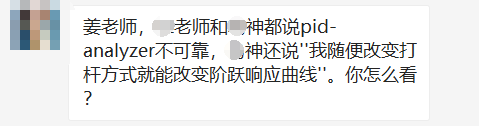我难以揣测这背后到底有着多少对于数学的恶意，也无法猜测这其中掺杂了多少不懂装懂的尴尬。
但是既然大家对这些工具的恶意这么大，索性今天也就不计字数，给大家粗略解释一下PID-Analyzer和PIDtoolbox的阶跃响应形状不同的原因，以及其背后的反卷积原理。
01
—
为什么同一个LOG会出现截然不同的结果？简单来说，这是因为
PID-Analyzer直接输出全部阶跃响应帧的平均结果，而
PIDtoolbox启用了根据稳定时长筛选阶跃响应帧的算法来控制分析数据的质量。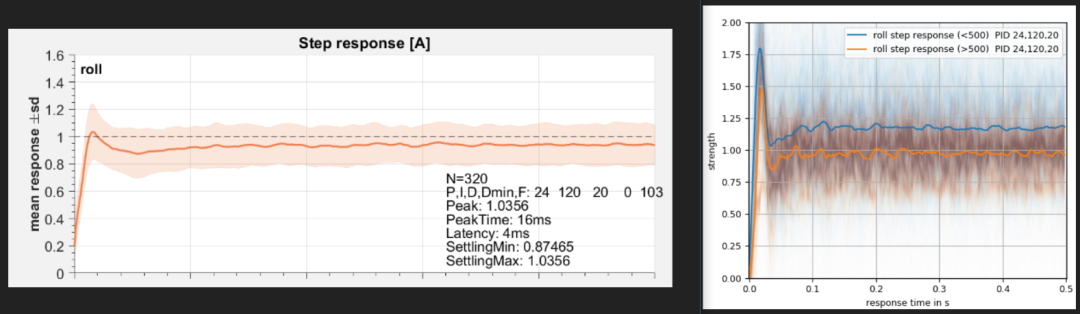在PIDtoolbox内看起来还算正常的log，在PID-analyzer里则完全超调查阅代码可知，PID-analyzer在进行维纳反卷积之后直接对全部阶跃响应帧进行了求平均，并进行了图像绘制。
def wiener_deconvolution(self, input, output, cutfreq):      # input/output are two-dimensional 输入输出信号均为二维信号  pad = 1024 - (len(input) % 1024)                        # padding to power of 2, increases transform speed 将 padding设置为2的幂次数以提高变换速度  input = np.pad(input, [[0,0],[0,pad]], mode='constant')    # 指定输入信号及模式，下为输出信号  output = np.pad(output, [[0, 0], [0, pad]], mode='constant')  H = np.fft.fft(input, axis=-1)  G = np.fft.fft(output,axis=-1)  freq = np.abs(np.fft.fftfreq(len(input), self.dt))  sn = self.to_mask(np.clip(np.abs(freq), cutfreq-1e-9, cutfreq))  len_lpf=np.sum(np.ones_like(sn)-sn)  sn=self.to_mask(gaussian_filter1d(sn,len_lpf/6.))  sn= 10.*(-sn+1.+1e-9)       # +1e-9 to prohibit 0/0 situations  Hcon = np.conj(H)  deconvolved_sm = np.real(np.fft.ifft(G * Hcon / (H * Hcon + 1./sn),axis=-1))  # 此为反卷积核心公式  return deconvolved_sm  # 将阶跃响应结果返回输出def stack_response(self, stacks, window):  # 绘制阶跃响应曲线  inp = stacks['input'] * window  outp = stacks['gyro'] * window  thr = stacks['throttle'] * window  deconvolved_sm = self.wiener_deconvolution(inp, outp, self.cutfreq)[:, :self.rlen]  delta_resp = deconvolved_sm.cumsum(axis=1)  max_thr = np.abs(np.abs(thr)).max(axis=1)  avr_in = np.abs(np.abs(inp)).mean(axis=1)  max_in = np.max(np.abs(inp), axis=1)  avr_t = stacks['time'].mean(axis=1)  return delta_resp, avr_t, avr_in, max_in, max_thr
摘自PID-Analyzer_v0.52.py而PIDtoolbox在完成反卷积之后，使用stepinfo()函数对阶跃响应质量进行了提取，并随后以稳定时间为指标对所有阶跃响应帧进行了筛选，以完成质量控制过程。如下所示。
j = 0；rateHigh = 0；for i=1:size(SPseg,1)  waitbar(i/size(SPseg,1),hw,['computing step response functions... ']);   a=fft(GYseg(i,:).*hamming(length(GYseg(i,:)))');% output, use hann or hamming taper  b=fft(SPseg(i,:).*hamming(length(SPseg(i,:)))');% input, use hann or hamming taper  G=a/length(a);  H=b/length(b);  Hcon=conj(H);       imp=real(ifft((G .* Hcon) ./ (H .* Hcon + .0001)))'; % impulse response function, .0001 to avoid divide by 0  impf =  smooth(imp, lograte*10); % minor smoothing  resptmp(i,:) = cumsum(impf); % integrate impulse resp functions  resptmp(i,:)=resptmp(i,:)-resptmp(i,1);  a=stepinfo(resptmp(i,1:wnd)); % gather info about quality of step resp function 抓取阶跃响应质量  if a.SettlingMin>.5 && a.SettlingMin<=1 && a.SettlingMax>1 && a.SettlingMax<2 %Quality control 质量控制过程    j=j+1;    stepresponse(j,:)=resptmp(i,1:1+wnd);     if max(abs(SPseg(i,:))) >= rateHighThreshold      rateHigh(j,:)=1;    else      rateHigh(j,:)=0;    end  end        t = 0:1/lograte:StepRespDuration_ms;% time in ms        end
摘自PIDtoolbox/PTstepcalc.m
也就是说，某些情况下，
对阶跃响应帧进行质量筛选，是导致同一段LOG在两个工具内跑出完全不一样结果的主要原因
。

02
—
那PIDtoolbox的结果还是正确的吗？部分是 - 前提是要理解为何我们能计算出阶跃响应。已知输入输出信号的时域曲线，将其变换到复频域后，使用(输出/输入)便可求得系统的传递函数，再将其变换回时域即可得冲激响应。对其积分即可得到阶跃响应。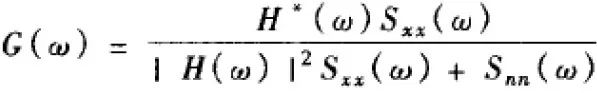这在数学上称为反卷积。反卷积是毫无对错可言的 - 它本来就是可推导的正确的数学定理。问题出现在质量控制部分。有一部分人认为系统的所有阶跃响应帧均可反映系统的实际性能，有一部分人则不这么认为。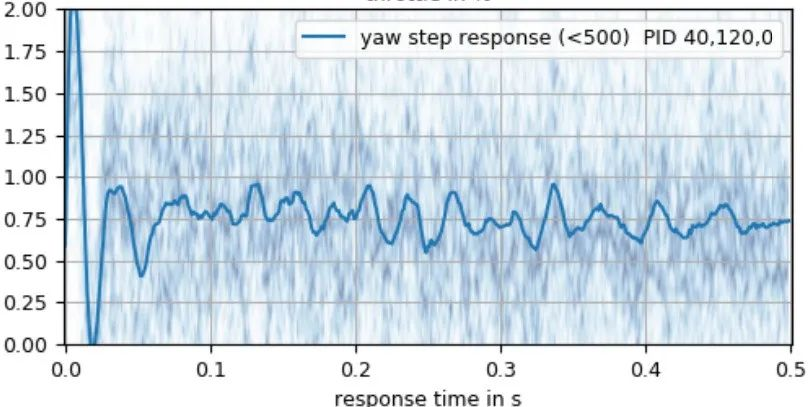认为
所有阶跃响应帧均可反映系统的实际性能的人的考虑点是这样的：系统的PIDF设计之初就是用来完成稳定问题和抗扰动问题，所以由于系统外非线性扰动造成的异常响应帧也应视为有效帧。洗桨、强风这些情况下的响应帧也应被计算在内。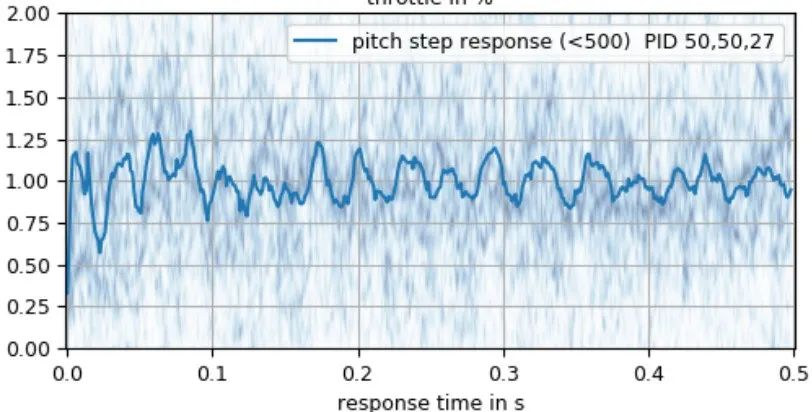另外一部分人认为，系统的设计前提是假定系统是局部线性，我们日常飞行也基本是处于局部线性段，而反卷积也是针对线性系统，所以我们应该只关注于局部线性部分的响应性能。而前馈确实会给系统带来一个相位超前的加速信号，这个信号会显著改变系统的阶跃响应曲线。所以，要分析系统自身的阶跃响应曲线，确实不应该使用前馈。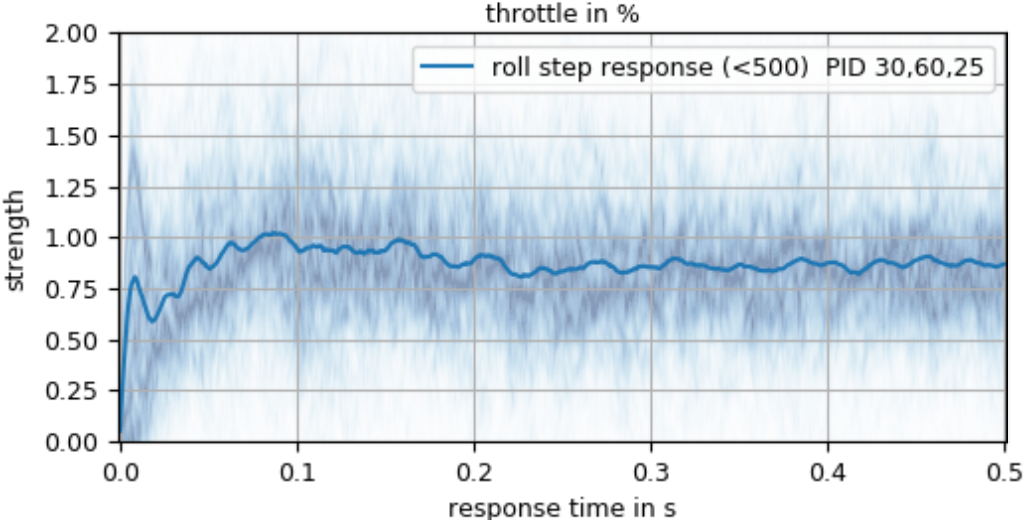无论如何，
反卷积算法在局部线性部分确实是科学有效的计算工具。但是，
穿越机是一个由线性系统和非线性系统相互耦合的复杂系统，只使用反卷积阶跃响应曲线法来作为判断PIDF参数的唯一判据显然是不正确的。
03
—
那么这种算法本身的可靠性如何？
要评估算法自身的可靠性，就要分析全部阶跃响应帧，就需要找到一种科学有效的方式来对阶跃响应帧进行归一化统计。
在数据分析领域，使用K-means平均算法来对信息进行聚类分析是常见的数据分析方法。下面我们便使用K-means对原始阶跃响应帧进行聚类分析。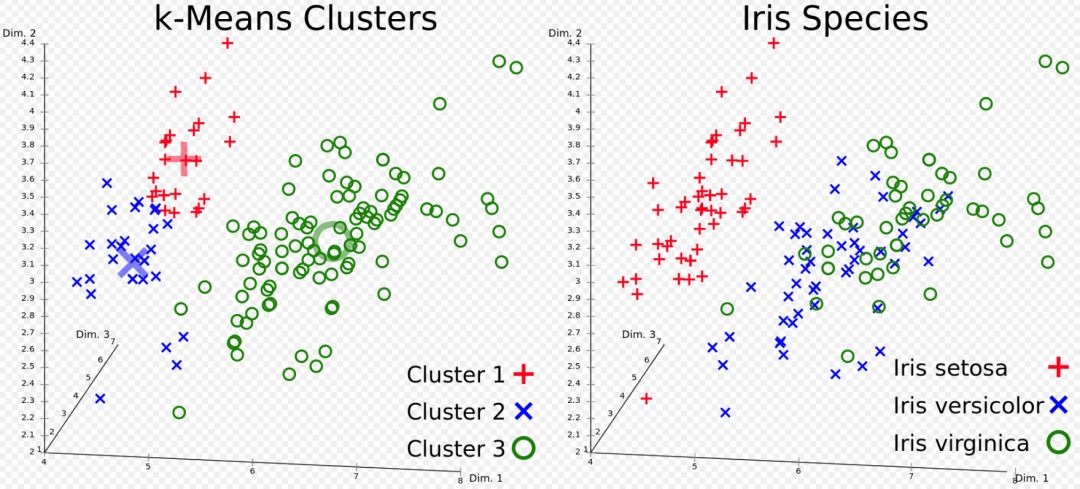图片源自K-means_clustering维基百科

第一步，打印出阶跃响应帧的数量。然后先对对数据进行白化(Whiten)处理，之后再调用Kmeans算法对其聚类，并在在欧几里得空间里标示出数据分布与聚类结果的质心位置。
step_response[j,:] = respprint("We now have %d different step responses" %j)from scipy.cluster.vq import vq,kmeans,whiten# Set the number of clusters# In my test, it seems that the best value is 6GROUP_COUNT = 6# Pass step resonse to featuresfeatures = step_response[0:j,:]# Whiten datawhitened = whiten(features)# Find N clusters in the datacodebook, distortion = kmeans(whitened, GROUP_COUNT)print("The distortion of the signal is" %distortion)print(distortion)result = vq(whitened, codebook)# Display centroids in Euclidean spaceplt.scatter(whitened[:,0],whitened[:,1])plt.scatter(codebook[:,0],codebook[:,1],c='r')plt.show()

然后，标示出各聚类结果对应的样本数量，这与PIDtoolbox的样本数量N的意义基本一致。在对阶跃响应帧进行处理时，同时统计输入信号(摇杆输入信号)，并在最终绘制图像时绘制与聚类后的阶跃响应曲线相对应的输入信号曲线，以判断摇杆输入信号对阶跃响应结果的影响。
# Display clustered step response curvesresp_cluster = np.zeros((GROUP_COUNT, segment_length))#print(resp_cluster)setpoint_cluster = np.zeros((GROUP_COUNT, segment_length))#print(setpoint_cluster)member_count = np.zeros(GROUP_COUNT)for i in range(j):    codebook_idx = result[i]    resp_cluster[codebook_idx] = resp_cluster[codebook_idx] + step_response[i]    setpoint_cluster[codebook_idx] = setpoint_cluster[codebook_idx] + input_segments[i]    member_count[result[i]] = member_count[result[i]] + 1for i in range(GROUP_COUNT):    plt.subplot(GROUP_COUNT,2,i*2+1)    plt.plot(resp_cluster[i]/member_count[i])    plt.subplot(GROUP_COUNT,2,i*2+2)    plt.plot(setpoint_cluster[i]/member_count[i])print(member_count)

通过对一段Betaflight 4.1的花飞LOG进行分析，产生如下结果。

其阶跃响应帧数量为1069个，聚类结果在欧几里得空间里分布的如下图所示，蓝点为白化后的分布点，红点为聚类结果质心。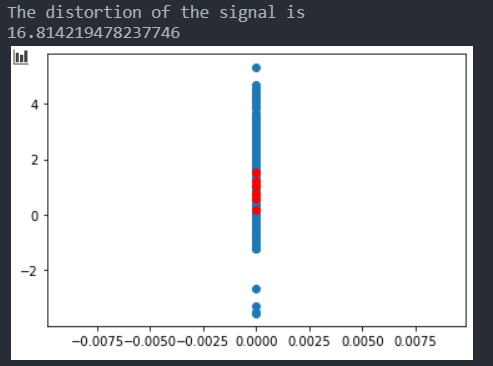聚类结果如下所示，其中左侧为阶跃响应曲线；右侧为与之对应的输入信号曲线；横轴2000代表样本数量，实际表示1秒的时间；自上到下的样本数量分别为469、15、398、6、109、72。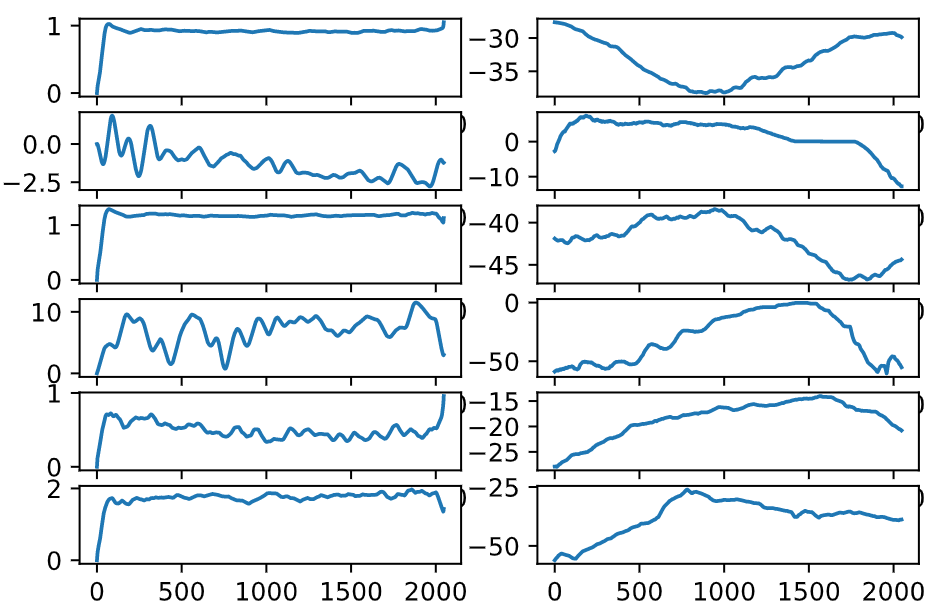与之对应，下面是此条LOG分别在PTB与PID-Analyzer内分析出来的阶跃响应曲线。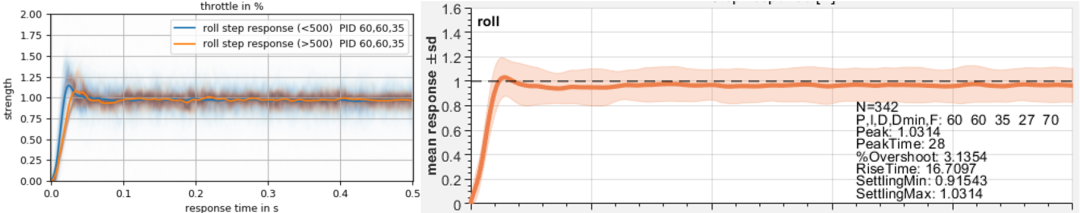可以看到，经K-means聚类后，样本数量为469与398的阶跃响应曲线与PTB大致相同。其样本数量最多，大致反应了飞行时的绝大多数情况下的阶跃响应曲线。
另外也可以看到，
“奇怪”的阶跃响应常发生于猛烈打杆后收杆归中时。我们应在飞行中尽量避免产生此类打杆操作。

04
—
结语
尽管K-means算法可以将阶跃响应进行聚类，产生一系列较为科学的结果，但仍需注意，K-means并不是一个稳定的算法，同一条LOG上可能会产生多种聚类结果。
虽然K-means的结果更加复杂难以直观判断，但是它却可以
将各类阶跃反应直观绘制出来。
经验丰富的技师可以根据聚类结果，对PIDF参数的适配程度有一个更完整的认知；执行能力强大的飞手可以根据聚类结果对应的摇杆输入情况，调整自己的打杆习惯，使飞行时飞机的动态范围总保持在局部线性区间内。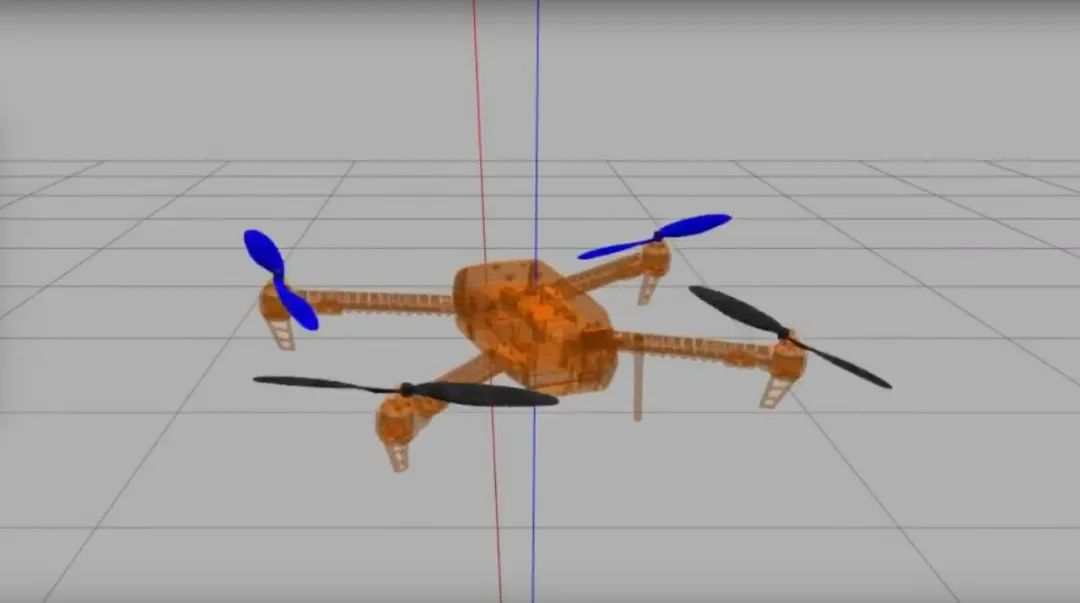图源GmyFC很抱歉将大家带入一个更加复杂的、尚未有确切定论的话题内，但我们的本意是打消大家对工具的算法的恶意。希望诸位读者在读完本文之后可以完成我们的初始目标(打消恶意)。
毕竟，真正的数学意义上的线性非线性并不是什么顺滑不顺滑；反卷积算法也不是什么仅仅理论可行实践掉链子的鸡肋算法；真正的PID阶跃响应结果也并不会如“大神”所言“我打个杆就能改变这条曲线”。但是切莫不可将任何一种算法的结果直接当成唯一的判据。正如本程序的幕后作者所言，
“使用K-Mean均值算法进行聚类只是一种判断干扰因素的手段，不是最终目的”。图文：市民姜先生
排版：SinCerely
程序：ColinNiu、市民姜先生
审核：ColinNiu、山鹰_SamFisher、SinCerely、辉光管
如需转载请后台留言
大神请勿直接抄袭
分享给朋友或朋友圈请随意哦

参考资料：
1. 反卷积和信号复原, 邹谋炎, 国防工业出版社
2. K-Mean-Cluster维基百科, https://en.wikipedia.org/wiki/K-means_clustering
3. PID-Analyzer源码
4. PIDtoolbox源码
5. pyPIDtoolbox源码


展开全文• . 电子科技大学中山学院学生实验报告 学院 机电工程学院 专业 17 ...MATLAB编程绘制控制系统的单位阶跃响应曲线 2 研究二阶控制系统中 n 对系统阶跃响应的影响 3 掌握准确读取动态特性指标的方法 4 分析二阶系统闭环极
• matlab阶跃响应曲线代码价钱 程序文件，“基于平稳国家定价的分布动力学”，西班牙银行工作文件0831，2008年12月，（C）James Costain和Anton Nakov 操作方法 运行“ gedyn.m”以计算一般平衡动力学； 冲激响应是...
• 二阶系统数学模型 标准形式的二阶系统结构图（来自《自动控制原理》第六版–胡寿松） 其中： 欠阻尼时的单位阶跃响应表达式为（图片来自教材）： 二阶系统欠阻尼单位阶跃响应 响应曲线如图所示，图中的右方的zeta为...
继上一篇博客 一阶系统时域分析，下面进行二阶系统时域分析，上篇链接：https://blog.csdn.net/qq_40035462/article/details/89350543
前言
在经典控制理论中，常用时域分析法、根轨迹法或频域分析法来分析线性控制系统的性能，不同的方法有不同的特点和适用范围，但是比较而言，时域分析法是一种直接在时间域中对系统进行分析的方法，具有直观、准确的优点，并且可以提供系统时间响应的全部信息。
 由于二阶系统情况较复杂，此处只实现了二阶系统在欠阻尼、无阻尼和临界阻尼情况下的阶跃响应。

二阶系统数学模型
标准形式的二阶系统结构图（来自《自动控制原理》第六版–胡寿松）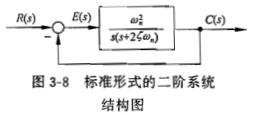其中：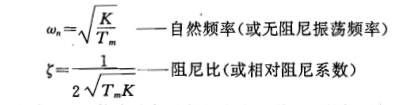欠阻尼时的单位阶跃响应表达式为（图片来自教材）：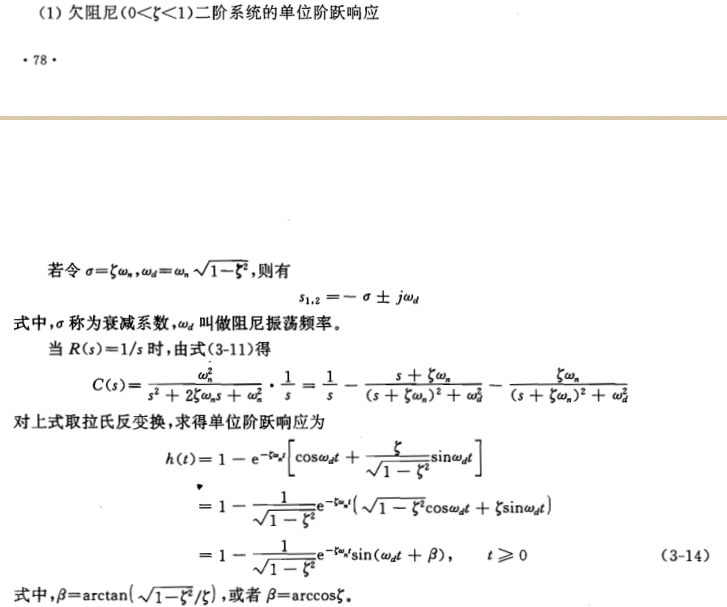二阶系统欠阻尼单位阶跃响应
响应曲线如图所示，图中的右方的zeta为阻尼比： 一共绘制了4条曲线，对应单位阶跃响应输入阻尼比为0（蓝色）、0.3（橙色）、0.7（绿色）、1（红色） 这四种情况，K为开环增益（图中均取值1），T为机电时间常数（取值1），Wn自然频率（取值1），图如下：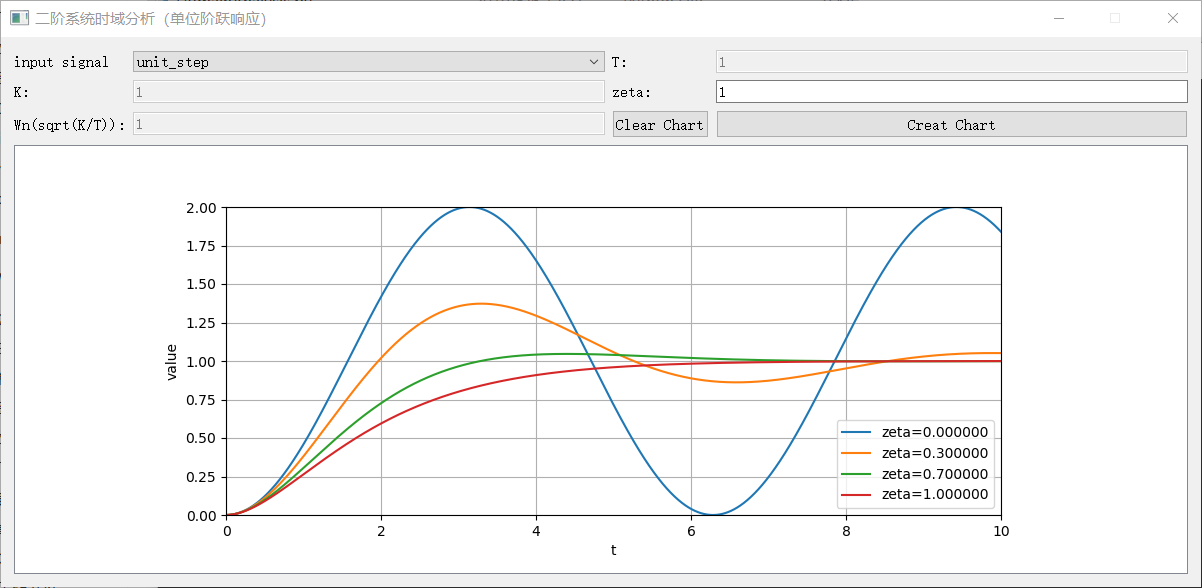当阻尼比为0.3，0.7时的动态性能指标（Tm，K，Wn均为1）如下：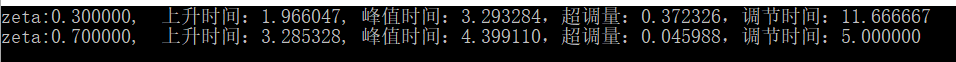由动态性能指标看出：阻尼比越小，上升时间越小，峰值时间越小，超调量越大，调节时间越大，与书本图形一致，书本图如下：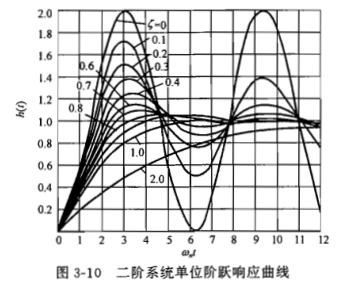展开全文自动控制原理
• 光伏电池阵列控制策略阶跃响应曲线，该曲线无超调，无震荡，所以此时的动态响应和稳态性能都达到了目标所期望的效果。DC/AC逆变器控制的是电压源，在此种情况下我们通常，采用双闭环控制方案。双闭环控制器是由两...
• matlab阶跃响应曲线代码第482章 目录 介绍 Furtua摆架也称为旋转倒立摆，是一个基本系统，可帮助举例说明非线性系统，该系统具有专门设计为可在水平面上绕z轴旋转的Sevro电机的旋转臂。 使用向心力，系统可以平衡...
• 电子科技大学中山学院学生实验报告 学院 机电工程学院 专业 17...MATLAB编程绘制控制系统的单位阶跃响应曲线 2 研究二阶控制系统中 n 对系统阶跃响应的影响 3 掌握准确读取动态特性指标的方法 4 分析二阶系统闭环极点和
• Transfer function: 1 ------- 5 s + 1 写成微分方程： 5y'(t)+y(t)=u(t) 向前差分： 5y(k+1)+(T-5)y(k)=Tu(k) T:Sample time 向后差分： ...Matlab阶跃响应： G1=tf(,[...


Transfer function:
1
-------
5 s + 1

写成微分方程：

5y'(t)+y(t)=u(t)

向前差分：

5y(k+1)+(T-5)y(k)=Tu(k)
T:Sample time

向后差分：

(5+t)y(k)-Ty(k-1)=Tu(k)

Matlab阶跃响应：

G1=tf(,[5 1]);
step(G1)      %连续传递函数阶跃响应曲线
hold on
G2=c2d(G1,0.1,'zoh');
step(G2)     %离散传递函数阶跃响应曲线
hold on
T=0.1;
G3=tf([T],[5 T-5],T);  %向前差分函数阶跃响应曲线
step(G3)
hold on
G4=tf([T],[5+T -T],T)
step(G4)             %向前差分函数阶跃响应曲线

转载于:https://www.cnblogs.com/conpi/p/11013031.html
展开全文• matlab阶跃响应曲线代码神经同步，以使用MiRo检测和分类触觉行为 MiRo是一种小型宠物式机器人，旨在作为伴侣。 在其头部和背部设有触觉传感器矩阵。 这些触觉传感器的值是通过基于ROS的用户通信通道获取的。 该任务...
• MATLAB中对象阶跃响应曲线的实现方法[参照].pdf软件开发
• 原创开发，使用Matlab求解阶跃响应指标(上升时间、调整时间、峰值峰值、超调量)，内含自定义Matlab函数、丰富的演示实例和详细的说明文档，可以方便求取系统阶跃响应指标，简单易用。
• 行业-电子政务-基于压电扫描管阶跃响应曲线的原子力显微镜动态成像方法.zip
• 项目背景：甘肃省，航天510所的LIPS100电推力器。一共有三个控制变量，...要求：比例控制参数P可调，阶跃响应在通电前设定幅值3.5~12.8 假设仿真实验时长120S，在第60S时给定阶跃控制信号。 把查表用的TXT文件...MATLAB
• 其中 wn＝2，阻尼比 分别为0.2，0.4，0.6，0.8，1，2时系统的单位阶跃响应曲线(绘制在同一张图上)，并求出 ＝2，阻尼比 ＝0.4时系统的峰值时间和超调量。   2 求解   2.1 将所有的单位阶跃响应绘制在一起 matlab ...
• 1.Matlab作单位阶跃响应曲线的三维图 定义闭环系统传递函数如下： H(s)=1/(s^2+2*zeta*s+1) %Matlab作单位阶跃响应曲线的三维图 %标准二阶系统响应曲线 wn=1 t=0:0.2:10; zeta=[0 0.2 0.4 0.8 1]; for n=...
• 用MATLAB求传递函数的单位阶跃响应并绘制出曲线matlab
• 专业:17自动化 课程名称自动控制原理实验与仿真 班级 姓名 学号组别 实验名称基于MATLAB勺控制系统单位阶跃响应分析 实验时间 成 绩 批改时间 一 实验目的 学会使用MATLABS程绘制控制系统的单位阶跃响应曲线 ......